# SSC CGL 2018 Practice Test Papers | Quantitative Aptitude (Day-2)

Dear Aspirants, Here we have given the Important SSC CGL Exam 2018 Practice Test Papers. Candidates those who are preparing for SSC CGL 2018 can practice these questions to get more confidence to Crack SSC CGL 2018 Examination.

[WpProQuiz 2609]

Click “Start Quiz” to attend these Questions and view Explanation

1. Refer the below diagram and answer the following questions.

In a class of 50 students, the number of students passed in Maths is represented as Circle and the number of students passed in English is represented as Rectangle and the number of students passed in Economics is represented as triangle.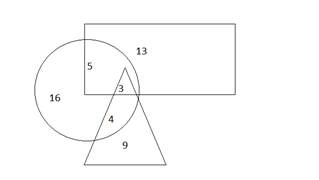What is the number of students passed in both Maths and English?

a.5

b.3

c.8

d.2

1. Refer the below diagram and answer the following questions.

In a class of 50 students, the number of students passed in Maths is represented as Circle and the number of students passed in English is represented as Rectangle and the number of students passed in Economics is represented as triangle.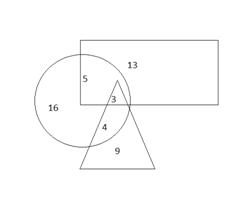What is the difference between the number of students passed in Maths alone and the number of students passed in all three subjects?

a.13

b.8

c.9

d.4

1. Refer the below diagram and answer the following questions.

In a class of 50 students, the number of students passed in Maths is represented as Circle and the number of students passed in English is represented as Rectangle and the number of students passed in Economics is represented as triangle.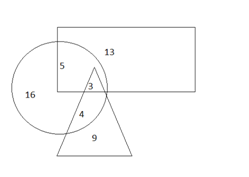If the number of students passed in English alone increased by 100% then what is the number of students passed in English?

a.34

b.24

c.26

d.30

1. Ragu and Ram ages are in the ratio 5:3. The sum of ages of Ragu, Ram and Ritesh is 81.

Ritesh age is half the difference between ages of Ragu and Ram. What is the age of Ram?

a.27

b.34

c.26

d.35

1. A line cuts the x ­axis at the point (­3, 0) and the y Axis at the point (0,6). What is the equation of the line?
1. x = 2y+6
2. y = 2x+6
3. x = 2y-6
4. y = -2x+6
1. A man can do a job in 80 hours. He works for 20 hours and then his efficiency reduced by 50%.How many additional hours does he take to complete the work?
1. 80
2. 50
3. 60
4. 160
1. The condition for two lines y1= m1x1+c1 and y2 = m2x2+c2 to be perpendicular is
1. m1=m2
2. m1=m2=0
3. m1m2=-1
4. m1m2=1
1. If P is 150% more than Q then find the value of and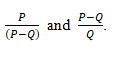a.3/5,5/2

b.5/3,3/2

c.2/3,3/2

d.2/3,5/3

1. Ratio of boys to girls in a school is 2:1. Ratio of passed boys and girls is 2:3. If 20% boys have passed, then what percentage of girls passed?
1. 40
2. 60
3. 50
4. 30
1. If (5x-3) = (4x+3), what is the value of (x+1)4?
1. 1096
2. 1024
3. 2401
4. 1331

Explanation:

5+3=8

Explanation:

16-3=13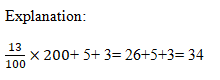Explanation:

Let the ages of Ragu and Ram be 5x and 3x respectively

Ritesh’s age = (5x – 3x) = x

5x+3x+x = 81

X = 9

3x = 3×9 = 27

Explanation: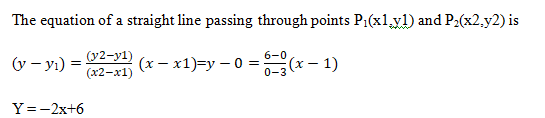6.Ans: c

Explanation:

20×+X1× = 1

X1=60

New efficiency,

20*1/80+X2*1/160 = 1

X2 = 120

Additional hours = x2-x1 = 120 – 60 = 60 hours

Explanation: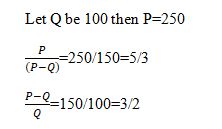Explanation:

Let B & G= 200 & 100

20 % of boys passed=40

No. of Girls passed= 40/2*3=60

Percentage of girls passed= 100/100*60=60

Explanation:

5x-3 = 4x+3

X = 6

(x+1)4= 7×7×7×7 = 2401